Please review your test results by moving cursor to answer sheet icon.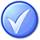0  (0%)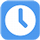6 Sec

1.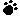Contingency Reserves are estimated costs to be used at the discretion of the project manager to deal with:

A) Inadequacies in the original estimate
B) Anticipated but not certain events.
C) Unanticipated events
D) Anticipated and certain events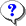Your answer=, Correct answer=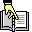, Work Sheet:

2.A project is estimated to cost \$ 50,000 with a timeline of 50 days. After 25 days, the project manager finds that 50% of the project is complete and Actual costs are \$ 50,000. What is the Cost Performance Index (CPI) ?

A) The CPI is 1
B) The CPI is 1.5
C) The CPI is 2
D) The CPI is 0.5Your answer=, Correct answer=, Work Sheet:

3.A variance threshold for costs or other indicators to indicate the agreed amount of variation allowed is called:

A) Variance limits
B) Control threshold
C) Cost limit
D) Acceptable thresholdYour answer=, Correct answer=, Work Sheet:

4.Lucy is a project manager involved in the Estimate Costs process in the initiation phase of a project. Given the limited detail available to her, what would you expect the range of her estimate to be and what would you call such an estimate?

A) -25 to +25 %, Rough Order of Magnitude
B) -10 to +10 %, Budgetary
C) -1 to +1 %, Definitive
D) -50 to +50 %, Rough Order of MagnitudeYour answer=, Correct answer=, Work Sheet:

5.An estimating technique that uses a statistical relationship between historical data and other variables (for example, square footage in construction, lines of code in software development) is known as:

A) Parametric Estimating
B) Analogous Estimating
C) Bottom-up Estimating
D) Historical AnalysisYour answer=, Correct answer=, Work Sheet:

 Click Turn Right Ahead sign for next test